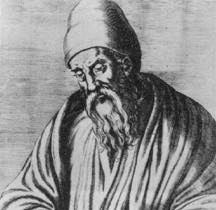# The story of Euclid and the infinitude of primes

Once upon a time, there lived a Mathematician named Euclid.Euclid came up with an ingenious method of proving that there are infinitely many prime numbers. Prime numbers are whole numbers that only have two factors, one and itself. For instance, 7 is a prime number while 6=2×3 is not.

The proof is by contradiction. Suppose that there are only a finite number of prime numbers,$p_1, p_2, \cdots, p_n$.

Now, we consider the number$P=p_1 \cdot p_2 \cdots p_n+1$.

P is either a prime number, or it is a composite number.

If P is a prime number, then we have just contradicted the assumption that there are only a finite number of prime numbers$p_1, p_2, \cdots, p_n$!

If P is a composite number, then, it has a factor (smaller than P). However, none of$p_1, p_2, \cdots, p_n$ can be a factor since P divided by those primes will leave a remainder of 1! Hence, P has another factor that is not in the original list, contradicting the initial assumption once again.

(Strictly speaking, Euclid’s proof is not by contradiction, instead he used a similar argument to show that given any finite list of primes, there is at least one other prime.)

As of now, the largest known prime to mankind is 257,885,161 − 1, a number with 17,425,170 digits! (http://en.wikipedia.org/wiki/Largest_known_prime_number)Euclid’s Elements## Author: mathtuition88

https://mathtuition88.com/

This site uses Akismet to reduce spam. Learn how your comment data is processed.